# Kmeans Clustering Kmeans Clustering What is clustering Why

• Slides: 18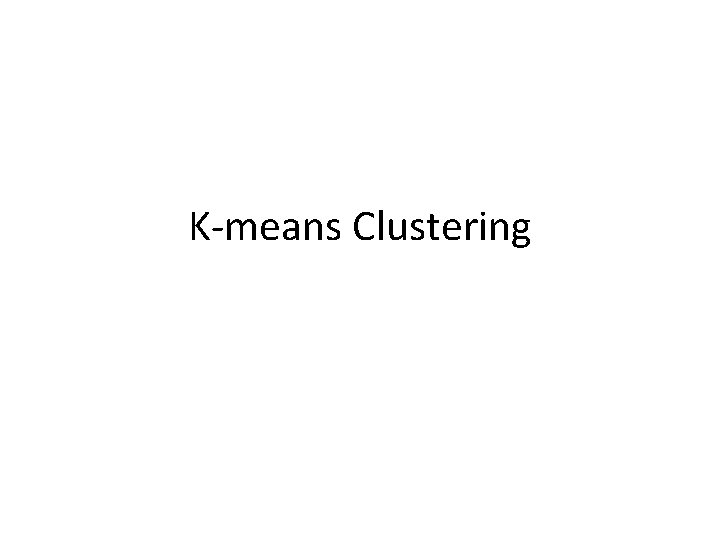K-means Clustering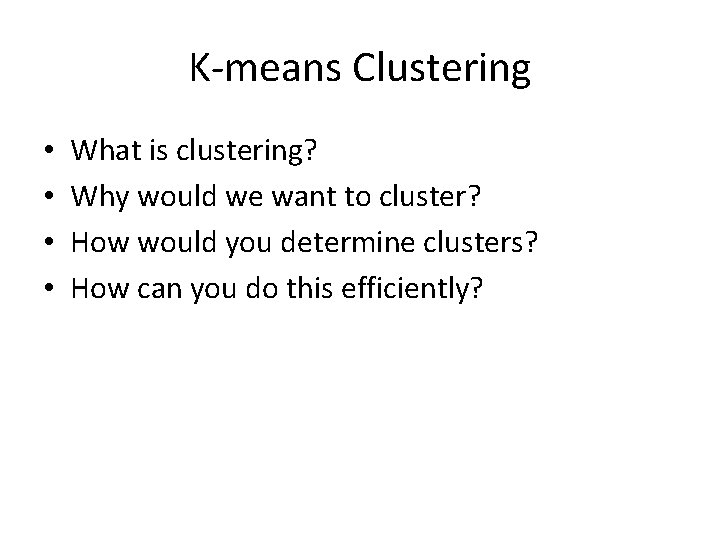K-means Clustering • • What is clustering? Why would we want to cluster? How would you determine clusters? How can you do this efficiently?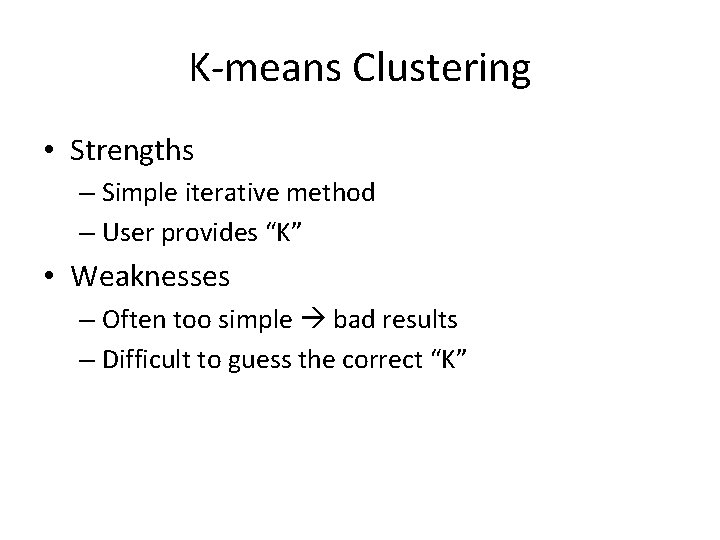K-means Clustering • Strengths – Simple iterative method – User provides “K” • Weaknesses – Often too simple bad results – Difficult to guess the correct “K”K-means Clustering Basic Algorithm: • Step 0: select K • Step 1: randomly select initial cluster seeds Seed 1 650 Seed 2 200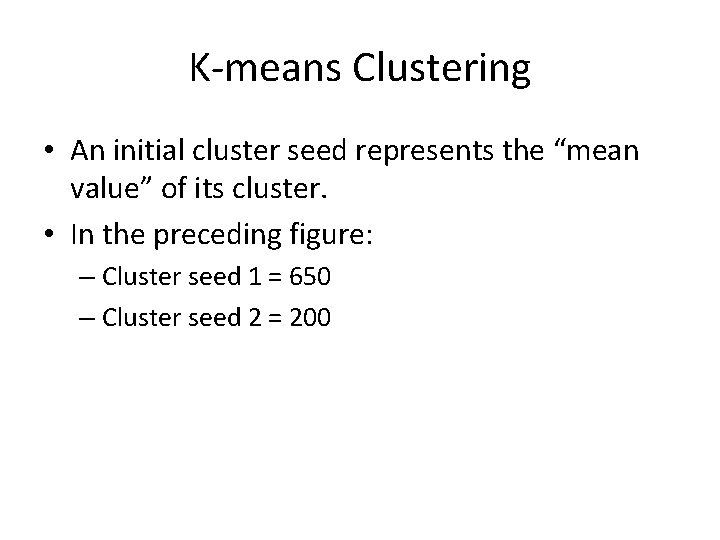K-means Clustering • An initial cluster seed represents the “mean value” of its cluster. • In the preceding figure: – Cluster seed 1 = 650 – Cluster seed 2 = 200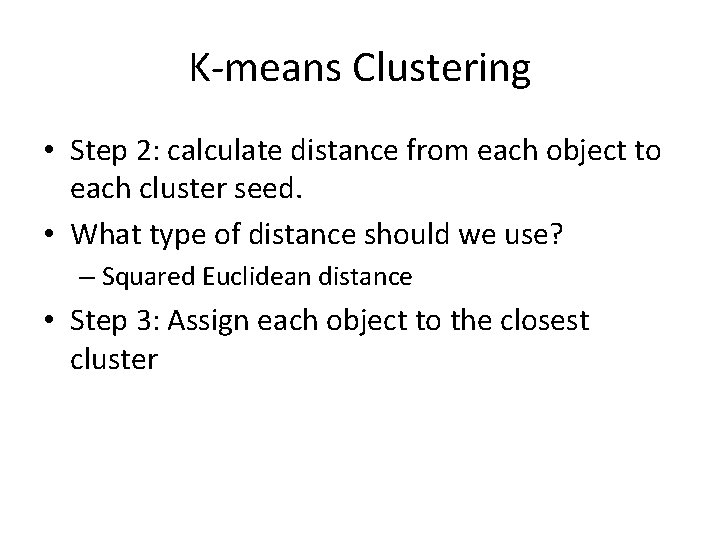K-means Clustering • Step 2: calculate distance from each object to each cluster seed. • What type of distance should we use? – Squared Euclidean distance • Step 3: Assign each object to the closest cluster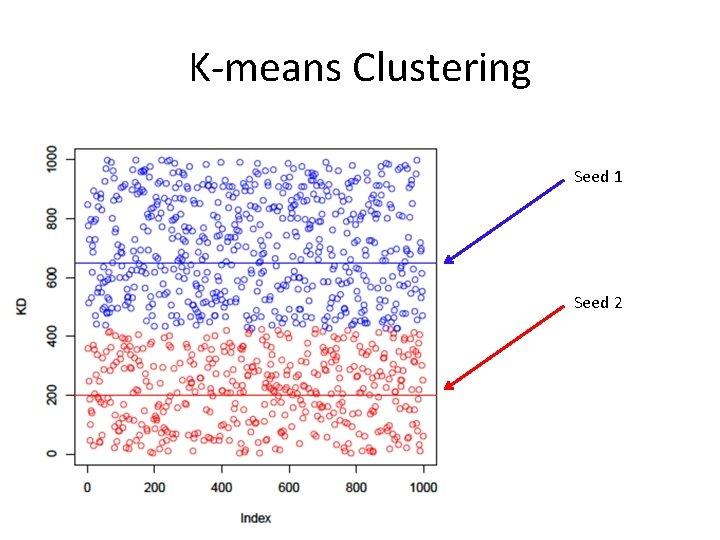K-means Clustering Seed 1 Seed 2K-means Clustering • Step 4: Compute the new centroid for each cluster Cluster Seed 1 708. 9 Cluster Seed 2 214. 2K-means Clustering • Iterate: – Calculate distance from objects to cluster centroids. – Assign objects to closest cluster – Recalculate new centroids • Stop based on convergence criteria – No change in clusters – Max iterationsK-means Issues • Distance measure is squared Euclidean – Scale should be similar in all dimensions • Rescale data? – Not good for nominal data. Why? • Approach tries to minimize the within-cluster sum of squares error (WCSS) – Implicit assumption that SSE is similar for each group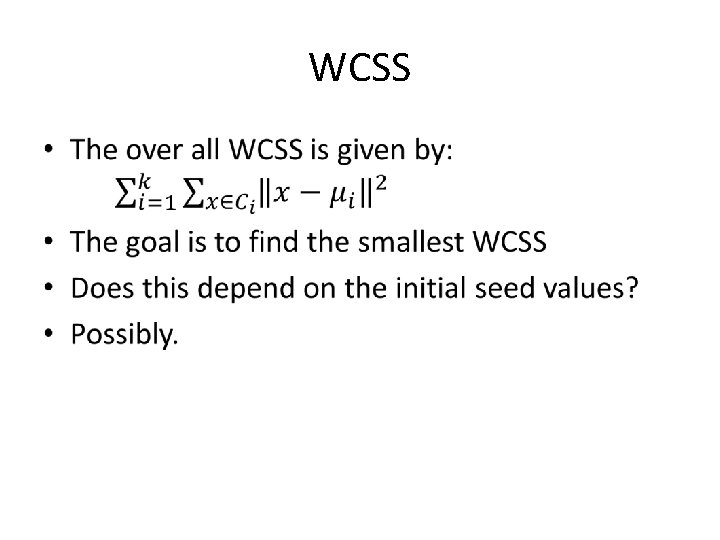WCSS •Bottom Line • K-means – Easy to use – Need to know K – May need to scale data – Good initial method • Local optima – No guarantee of optimal solution – Repeat with different starting valuesK-Means Lab Pause this set of slides and switch to lab slidesOther Clustering in R • Hierarchical Clustering (agglomerative) – Create a distance matrix using ‘dist()’ – Create the hierarchy using ‘hclust()’ • Model Based Clustering – Use ‘mclust()’ to create the clusters on the basis of • Bayesian Information Criterion (BIC) • Parameterized Gaussian mixture modelsHierarchical Clustering # Create the distance matrix d <- dist(state_income\$V 2, method = "euclidean") # Create the hierarchy fit <- hclust(d, method="ward. D 2") # Plot the histogram plot(fit) # cut the tree into 6 clusters Groups <- cutree(fit, k=6) # Outline the 6 clusters rect. hclust(fit, k=6, border="red")Hierarchical Clustering # Other hierarchical methods sfit <- hclust(d, method="single") cfit <- hclust(d, method="complete") afit <- hclust(d, method="average") # Plot the histogram op <- par(mar = c(0, 4, 4, 2), mfrow = c(2, 2)) plot(sfit, labels = FALSE, main = "Single", xlab = "") plot(cfit, labels = FALSE, main = "Complete", xlab = "") plot(afit, labels = FALSE, main = "Average", xlab = "") plot(fit, labels = FALSE, main = "Ward", xlab = "")Model-Based Clusters This is more complicated that hierarchical or K-means clustering # First load mclust package #Create the cluster(s) fit <- Mclust(state_income\$V 2) # examine the result(s) summary(fit) # plot the result(s) plot(fit) #Not very satisfying. Try a different data set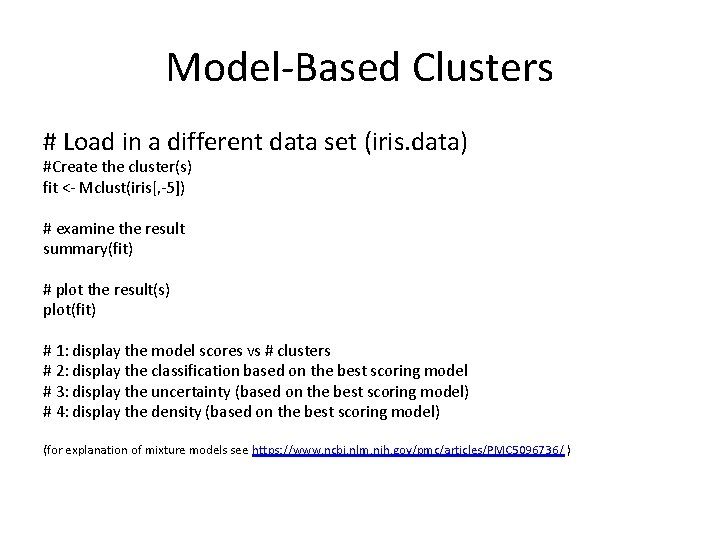Model-Based Clusters # Load in a different data set (iris. data) #Create the cluster(s) fit <- Mclust(iris[, -5]) # examine the result summary(fit) # plot the result(s) plot(fit) # 1: display the model scores vs # clusters # 2: display the classification based on the best scoring model # 3: display the uncertainty (based on the best scoring model) # 4: display the density (based on the best scoring model) (for explanation of mixture models see https: //www. ncbi. nlm. nih. gov/pmc/articles/PMC 5096736/ )